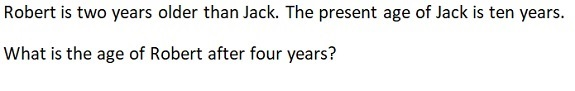Latest Results:

# 11plus Ages sample papers

Solve this Question

####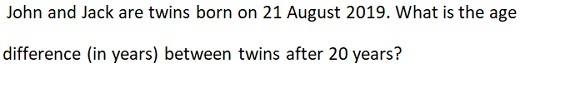Solve this Question

####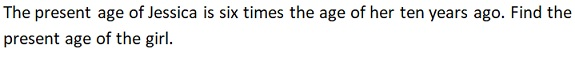1. 8 2. 6 3. 12 4. 24

Solve this Question

####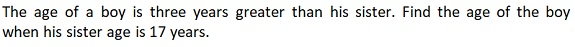1. 14 2. 15 3. 21 4. 20

Solve this Question

####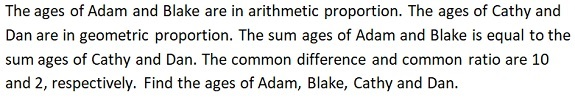1. Adam’s age = 20, Blake’s age = 10; Cathy’s age = 20; Dan’s age = 10 2. Adam’s age = 12, Blake’s age = 20; Cathy’s age = 12; Dan’s age = 20 3. Adam’s age = 10, Blake’s age = 20; Cathy’s age = 10; Dan’s age = 20 4. Adam’s age = 8, Blake’s age = 20; Cathy’s age = 8; Dan’s age = 20

####